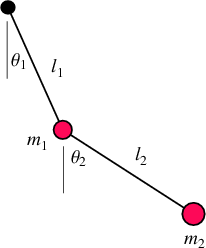[This article was first published on schochastics, and kindly contributed to R-bloggers]. (You can report issue about the content on this page here)
Want to share your content on R-bloggers? click here if you have a blog, or here if you don't.

This post is dedicated to the beautiful chaos created by double pendulums. I have seen a great variety of animated versions, implemented with different tool but never in R. Thanks to the amazing package gganimate, it is actually not that hard to produce them in R.library(tidyverse)
library(gganimate)

I am not going to attempt to explain the math behind the double pendulum. If you are interested in the details, check out a complete walkthrough here. The code presented here is a straightforward adaption from python.

First, we need to set up some basic constants and the starting conditions.

# constants
G  <-  9.807  # acceleration due to gravity, in m/s^2
L1 <-  1.0    # length of pendulum 1 (m)
L2 <-  1.0    # length of pendulum 2 (m)
M1 <-  1.0    # mass of pendulum 1 (kg)
M2 <-  1.0    # mass of pendulum 2 (kg)

parms <- c(L1,L2,M1,M2,G)

# initial conditions
th1 <-  20.0  # initial angle theta of pendulum 1 (degree)
w1  <-  0.0   # initial angular velocity of pendulum 1 (degrees per second)
th2 <-  180.0 # initial angle theta of pendulum 2 (degree)
w2  <-  0.0   # initial angular velocity of pendulum 2 (degrees per second)

state <- c(th1, w1, th2, w2)*pi/180  #convert degree to radians

These are the parameters you need to change in order to produce different pendulums. Just experiment a little!

The partial derivatives needed can be calculated with the following function.

derivs <- function(state, t){
L1 <- parms
L2 <- parms
M1 <- parms
M2 <- parms
G  <- parms

dydx    <-  rep(0,length(state))
dydx <-  state

del_ <-  state - state
den1 <-  (M1 + M2)*L1 - M2*L1*cos(del_)*cos(del_)
dydx  <-  (M2*L1*state*state*sin(del_)*cos(del_) +
M2*G*sin(state)*cos(del_) +
M2*L2*state*state*sin(del_) -
(M1 + M2)*G*sin(state))/den1

dydx <-  state

den2 <-  (L2/L1)*den1
dydx  <-  (-M2*L2*state*state*sin(del_)*cos(del_) +
(M1 + M2)*G*sin(state)*cos(del_) -
(M1 + M2)*L1*state*state*sin(del_) -
(M1 + M2)*G*sin(state))/den2

return(dydx)
}

This function needs to be integrated. Luckily, there is the odeintr packages that does the job. The start, duration and step_size parameters control the time for your pendulum. In the below example, I choose to “swing” the pendulum for 30 seconds and the position is recalculated every 0.1 seconds.

sol <- odeintr::integrate_sys(derivs,init = state,duration = 30,
start = 0,step_size = 0.1)

Now we just need to compute the x and y coordinates for both pendulums from the angles $$\theta$$ obtained from the integration.

x1 <-  L1*sin(sol[, 2])
y1 <-  -L1*cos(sol[, 2])

x2 <- L2*sin(sol[, 4]) + x1
y2 <- -L2*cos(sol[, 4]) + y1

df <- tibble(t=sol[,1],x1,y1,x2,y2,group=1)

The final data frame contains the exact position of the pendulums for each time step. Animating the pendulums is straightforward with the package gganimate.

ggplot(df)+
geom_segment(aes(xend=x1,yend=y1),x=0,y=0)+
geom_segment(aes(xend=x2,yend=y2,x=x1,y=y1))+
geom_point(size=5,x=0,y=0)+
geom_point(aes(x1,y1),col="red",size=M1)+
geom_point(aes(x2,y2),col="blue",size=M2)+
scale_y_continuous(limits=c(-2,2))+
scale_x_continuous(limits=c(-2,2))+
ggraph::theme_graph()+
labs(title="{frame_time} s")+
transition_time(t) -> p

pa <- animate(p,nframes=nrow(df),fps=20)
paWe can also add some more details to the animation, like the trail of the second pendulum to track its path. It turned out to be a bit more tricky then expected though. The trail needs to be added via a secondary data.frame so that it can be animated with the transition_time(). I used the lag() function to compute the trail from the last five time points.

tmp <- select(df,t,x2,y2)
trail <- tibble(x=c(sapply(1:5,function(x) lag(tmp$x2,x))), y=c(sapply(1:5,function(x) lag(tmp$y2,x))),
t=rep(tmp\$t,5)) %>%
dplyr::filter(!is.na(x))

I used the shadow_mark() function to keep the past trails.

ggplot(df)+
geom_path(data=trail,aes(x,y),colour="blue",size=0.5)+
geom_segment(aes(xend=x1,yend=y1),x=0,y=0)+
geom_segment(aes(xend=x2,yend=y2,x=x1,y=y1))+
geom_point(size=5,x=0,y=0)+
geom_point(aes(x1,y1),col="red",size=M1)+
geom_point(aes(x2,y2),col="blue",size=M2)+
scale_y_continuous(limits=c(-2,2))+
scale_x_continuous(limits=c(-2,2))+
ggraph::theme_graph()+
labs(title="{frame_time} s")+
transition_time(t)+
shadow_mark(colour="grey",size=0.1,exclude_layer = 2:6)-> p

pa <- animate(p,nframes=nrow(df),fps=20)
paThat’s it. Now you can play around with the constants (L1,L2,M1,M2,G) and the initial conditions (th1,w1,th2,w2) to create your own chaotic pendulums.

Below is my personal favorite. 40 pendulums with nearly identical starting conditions. Watch how quickly their paths diverge into pure chaos.